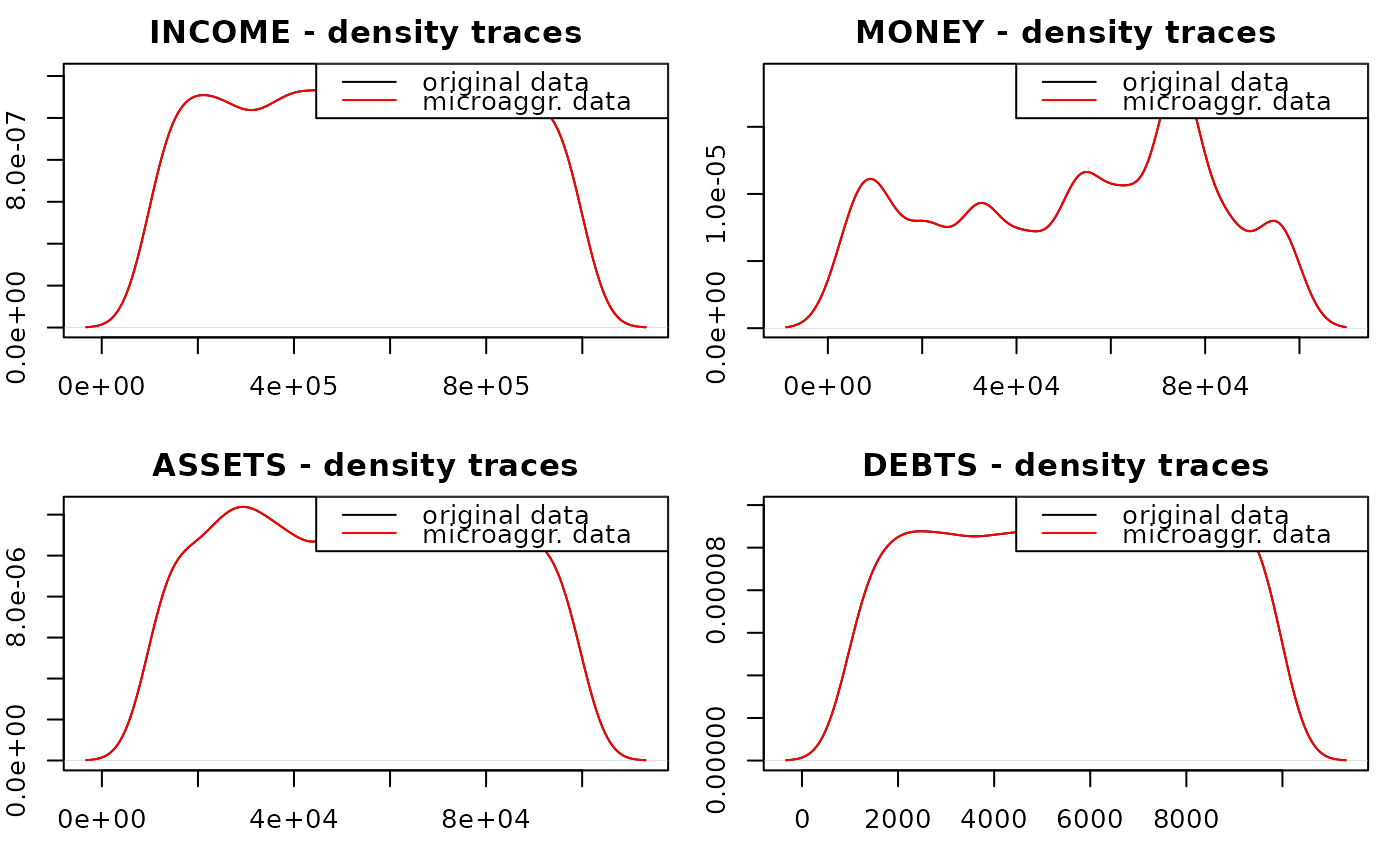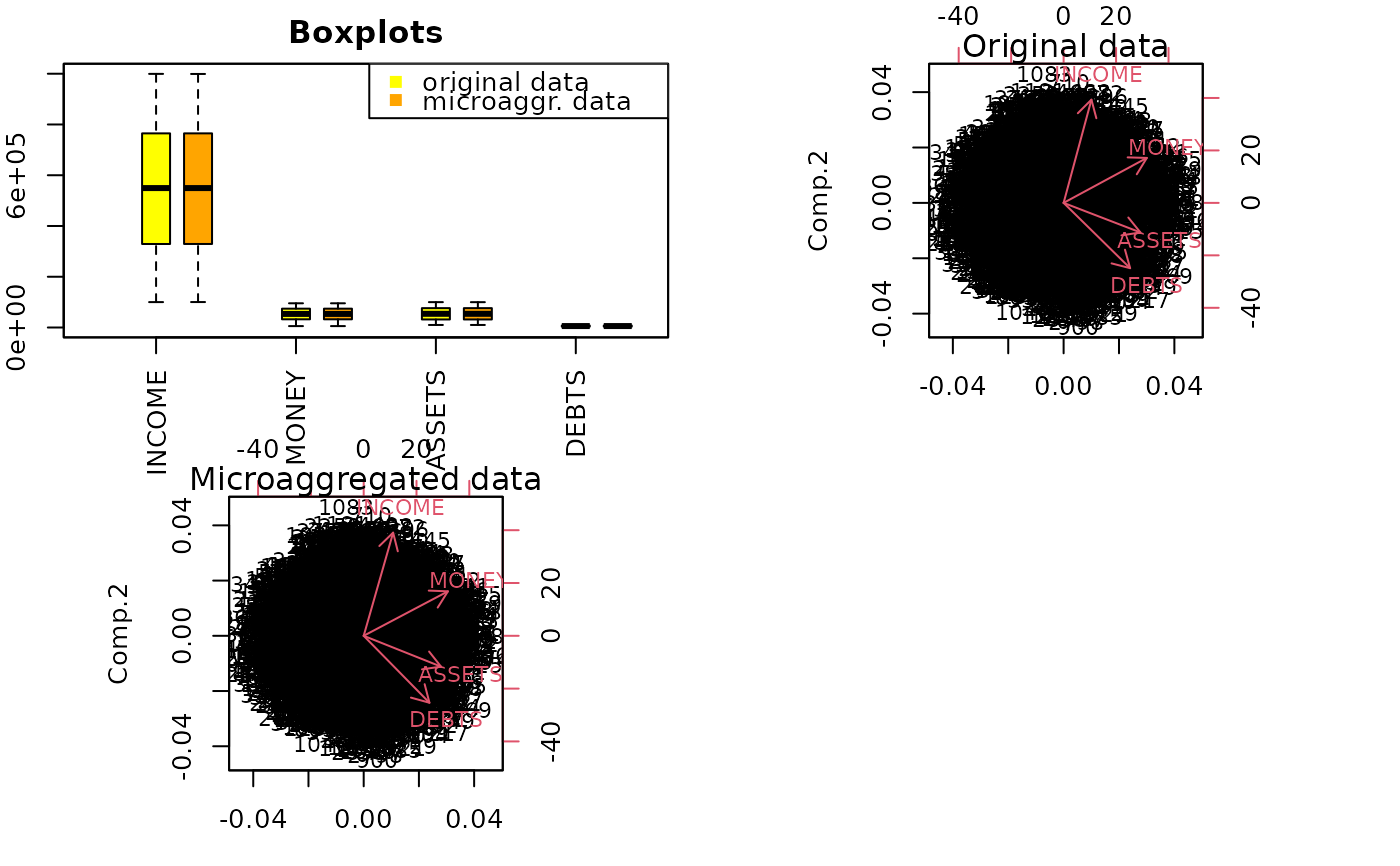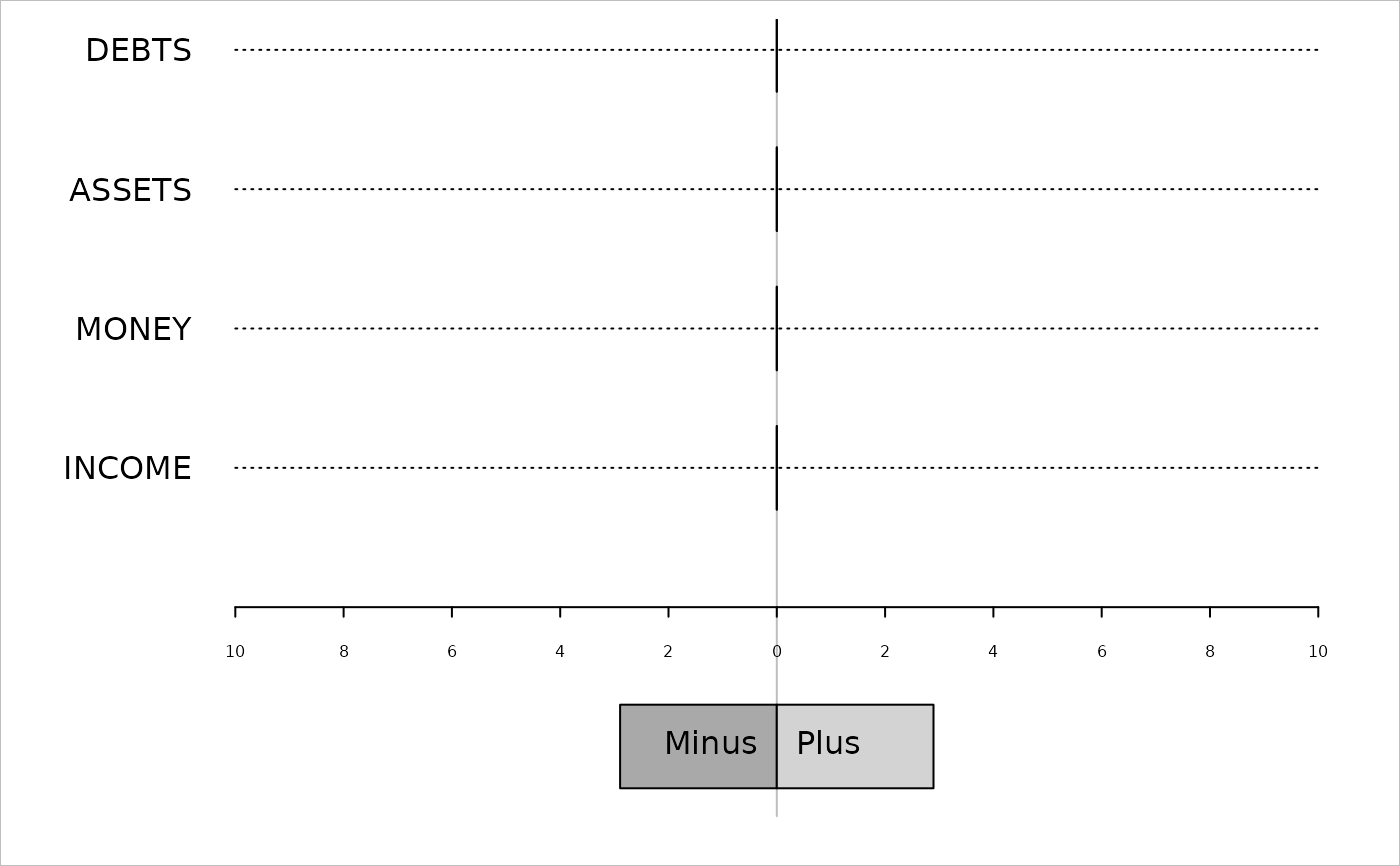Plots for the comparison of the original data and perturbed data.

plotMicro(x, p, which.plot = 1:3)

## Arguments

x

an output object of microaggregation()

p

necessary parameter for the box cox transformation (lambda)

which.plot

which plot should be created?

• 1: density traces

• 2: parallel boxplots

• 3: differences in totals

## Value

returns NULL; the selected plot is displayed

## Details

Univariate and multivariate comparison plots are implemented to detect differences between the perturbed and the original data, but also to compare perturbed data which are produced by different methods.

microaggregation()

Matthias Templ

## Examples

data(free1)
df <- as.data.frame(free1)[, 31:34]
m1 <- microaggregation(df, method = "onedims", aggr = 3)
plotMicro(m1, p = 1, which.plot = 1)plotMicro(m1, p = 1, which.plot = 2)plotMicro(m1, p = 1, which.plot = 3)